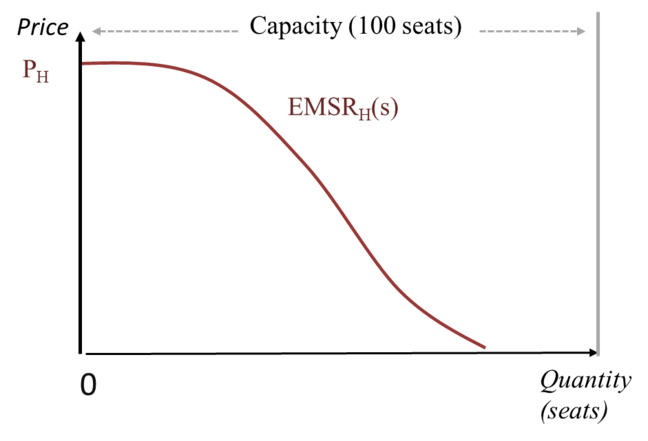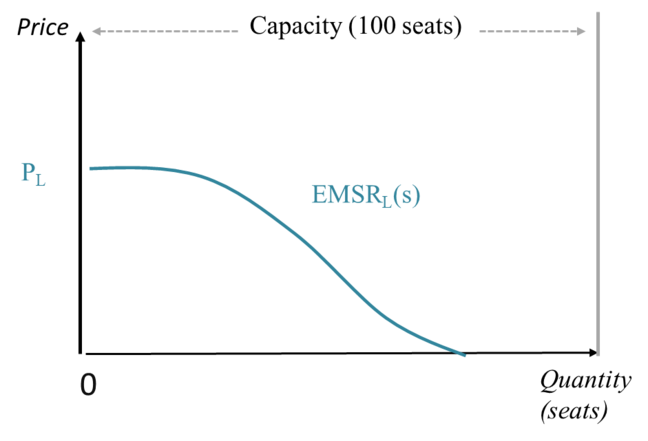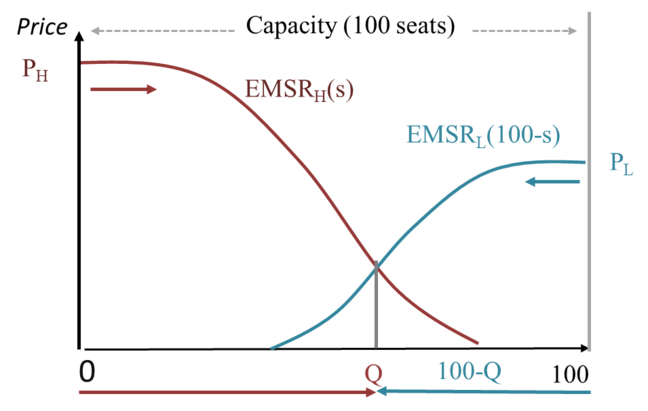## Want to keep learning?

This content is taken from the École Nationale de l'Aviation Civile's online course, An Introduction to Pricing Strategy and Revenue Management. Join the course to learn more.
3.7

# A key notion: The Expected Marginal Seat Revenue (EMSR)

The notion of Expected Marginal Seat Revenue (EMSR) is central to revenue management.

It can be adapted to things other than seats – rooms, cabins, spots, tables – but will always rely on expectations and, therefore, probabilities.

The $$EMSR$$ for the seat $$s$$ is defined as the price multiplied by the probability of selling an additional seat. We can also understand the $$EMSR(s)$$ as the expected value of the current seat $$s$$.

However, this value depends both on the price and on the probability of sales.

Let us recall the example of a boat with 100 similar seats sold either at high ($$P_H$$) or low ($$P_L$$) price.

In the following figure, we plot the Expected Marginal Seat Revenue for the higher priced seat, $$EMSR_H(s)$$, as a function of the number of seats sold.

Note that you cannot expect the value for any seat to be greater than its price. So, the $$EMSR_H$$ of any seat lies between its price, $$P_H$$, (if you are certain to sell it) and $$0$$ (if there is no chance at all of selling it).

The construction of the curve starts on the left. Since the first seat is quite likely to be sold, the probability of selling it is close to $$1$$ and the $$EMSR$$ of the first seat is close to the high price $$P_H$$. The probability of selling the second seat is lower, so the expected value of the second seat is lower.

The $$EMSR$$ curve decreases towards zero. At some point, it becomes improbable to sell any additional seats!The same reasoning applies to the expected value of a seat sold at the lower price, $$P_L$$.Note that both curves show the expected values of seats, one curve for the seats sold at the higher price and the other for the seats sold at the lower price. Those values are comparable, and we want to find the optimal number of seats to allocate at the higher price, $$Q$$. This is referred to as the optimal quota of seats to protect at the higher price.

$$Q$$ should be such that the expected value of the last seat sold at the higher price is (nearly) equal to the corresponding expected value for the remaining seats at the lower price.

To find that optimal quota graphically, we use a simple trick and flip the curve representing the $$EMSR_L$$ at the lower price horizontally. So, for the $$EMSR_L$$, the horizontal axis starts on the right, as presented below.At the intersection of the curves, the expected value of selling a seat at high and low price is the same. A company should seek to protect $$Q$$ seats at the higher price and offer $$100 – Q$$ seats at the lower price, if the total capacity is 100. This quota, $$Q$$, corresponds to the optimal trade-off between spoilage and spill.

## To sum up

Even in this simple setting with only two prices, the decision on the number of seats to protect at the higher price depends on a precise knowledge of each demand.

Knowing the average demand is not sufficient. What is important to know is not the average number of seats one sells, but the probability of selling more than a threshold (whether that’s 50 seats, 60 or even 70 seats). If the company has collected data on past bookings, those probabilities are quite easy to calculate, and so is the $$EMSR$$!

This calculation has to be done for both demands such that the optimal quota can be determined, either graphically or analytically.